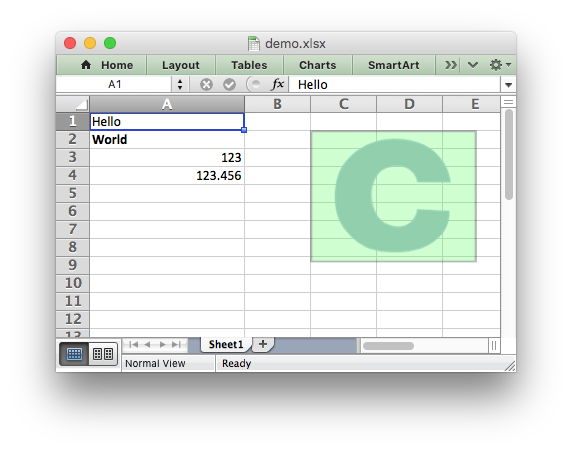libxlsxwriter
demo.c

A simple example of some of the features of the libxlsxwriter library./*
* A simple example of some of the features of the libxlsxwriter library.
*
* Copyright 2014-2021, John McNamara, jmcnamara@cpan.org
*
*/
#include "xlsxwriter.h"
int main() {
/* Create a new workbook and add a worksheet. */
lxw_workbook *workbook = workbook_new("demo.xlsx");
/* Set the bold property for the format */
format_set_bold(format);
/* Change the column width for clarity. */
worksheet_set_column(worksheet, 0, 0, 20, NULL);
/* Write some simple text. */
worksheet_write_string(worksheet, 0, 0, "Hello", NULL);
/* Text with formatting. */
worksheet_write_string(worksheet, 1, 0, "World", format);
/* Write some numbers. */
worksheet_write_number(worksheet, 2, 0, 123, NULL);
worksheet_write_number(worksheet, 3, 0, 123.456, NULL);
/* Insert an image. */
worksheet_insert_image(worksheet, 1, 2, "logo.png");
workbook_close(workbook);
return 0;
}
workbook_close
lxw_error workbook_close(lxw_workbook *workbook)
Close the Workbook object and write the XLSX file.
workbook_new
lxw_workbook * workbook_new(const char *filename)
Create a new workbook object.
format_set_bold
void format_set_bold(lxw_format *format)
Turn on bold for the format font.
worksheet_insert_image
lxw_error worksheet_insert_image(lxw_worksheet *worksheet, lxw_row_t row, lxw_col_t col, const char *filename)
Insert an image in a worksheet cell.
lxw_worksheet
Struct to represent an Excel worksheet.
Definition: worksheet.h:2107
lxw_format
Struct to represent the formatting properties of an Excel format.
Definition: format.h:358
lxw_workbook
Struct to represent an Excel workbook.
Definition: workbook.h:292
worksheet_write_string
lxw_error worksheet_write_string(lxw_worksheet *worksheet, lxw_row_t row, lxw_col_t col, const char *string, lxw_format *format)
Write a string to a worksheet cell.
worksheet_write_number
lxw_error worksheet_write_number(lxw_worksheet *worksheet, lxw_row_t row, lxw_col_t col, double number, lxw_format *format)
Write a number to a worksheet cell.
worksheet_set_column
lxw_error worksheet_set_column(lxw_worksheet *worksheet, lxw_col_t first_col, lxw_col_t last_col, double width, lxw_format *format)
Set the properties for one or more columns of cells.